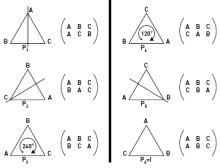# SC 2016 Semilab: Groups Around Us

by Pavel Etingof"Algebraists usually define groups as sets with operations satisfying a long list of difficult-to-remember axioms. To my mind, such a definition is impossible to understand. I think that algebraists put such obstacles on the way of those wishing to study their subject to make it more difficult for the uninitiated to get into, and thereby to raise their authority."
(V. I. Arnold, "Mathematics with a human face", "Priroda", 1988)

The notion of a group serves for a mathematical description of
symmetry (see http://en.wikipedia.org/wiki/Group_theory). For this reason, groups surround us everywhere. The goal of this course is to become familiar with the notion of a group and numerous examples of groups, and to learn to see and use groups in the world around us. It should be accessible to high school students interested in mathematics.

Plan of the course:

1. Transformation groups. Examples: groups of rotations, symmetries, motions.

2. Composition of permutations. The symmetric group.

3. Abstract groups (sets with operations satisfying a long list of difficult-to-remember axioms). Abelian (commutative) groups. Subgroups. Actions of a group. Realization of a finite group as a subgroup of the symmetric group. How to draw a graph whose group of symmetries is any finite group? Lagrange's theorem (the number of elements in a group is divisible by the number of elements in its subgroup). Index of a subgroup. Applications to combinatorics (the number of arrangements, combinations, labelled graphs).

4. More examples: integers and other numerical groups. Cyclic groups, cyclicity of groups of prime order, multiplication of matrices. How many sets are there if you put all the "Set" cards on the table?

5. Homomorphisms. Kernel and image. Normal subgroups and quotient groups. Direct and semidirect product. Examples (groups of small orders). Classification of finitely generated abelian groups (without proof).

6. Classification of finite groups of rotations of the 3-space and of regular polytopes.

7. Crystallographic groups in the plane (types of ornaments, or Frieze patterns) and in space (types of crystals).

8. Free groups and their universal property. Applications of groups to topology: fundamental groups. The goat problem. Fundamental groups of graphs and surfaces. Why is a subgroup of a free group free?

9. Groups defined by generators and relations. Can a computer check if a given element of a group equals 1? Homophonic quotients of free groups:
http://www.emis.de/journals/EM/restricted/2/2.3/mestre.ps
(a joke paper).

10. The group of symmetries of the Rubik cube.

11. Finite simple groups. The Jordan-Holder theorem (any finite group "decomposes" into simple ones.

12. The classification of finite simple groups (with a complete proof):
http://en.wikipedia.org/wiki/Classification_of_finite_simple_groups

13. (Devil's dozen) An exam on item 12.

The materials of the course can be found here:

http://www-math.mit.edu/~etingof/groups.pdf

Back to the list of semilabs